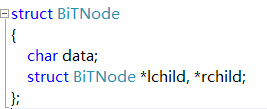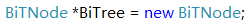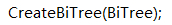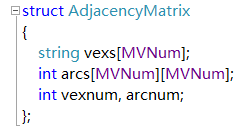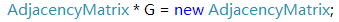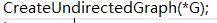---------------------------------问题补充--------------------------------------------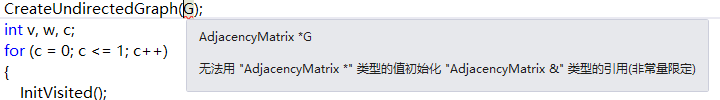2个回答

1. 值传递：形参是实参的拷贝，改变形参的值并不会影响外部实参的值。从被调用函数的角度来说，值传递是单向的（实参->形参），参数的值只能传入，不能传出。当函数内部需要修改参数，并且不希望这个改变影响调用者时，采用值传递。
2.指针传递一般适用于：函数内部修改参数并且希望改动影响调用者。对比值传递，指针传递可以将改变由形参“传给”实参（实际上就是直接在实参的内存上修改，不像值传递将实参的值拷贝到另外的内存地址中才修改）。C语言中函数调用参数的问题......
for (ctr = 0; ctr <= numMov; ctr++) { printf("请输入电影的名字\n\n"); scanf_s("%c", namMov); printf("请对该电影进行评分！\n\n"); scanf("%d", &rating); if (rating>fisRating) { strcpy_s(favorite, namMov); -- 程序在此指出错误：函数调用中的参数太少 fisRating = rating; 请问应该怎么解决？？？

![图片说明](https://img-ask.csdn.net/upload/202003/26/1585225574_353637.png) /*7.定义一个点类Point，包括x坐标和y坐标（int）。 定义一个CRect类，代表一个矩形， 要求CRect类中有代表矩形的左上角坐标（x1,y1） 和右下角坐标（x2,y2）点类的对象， 要求CRect类中有两个成员函数RectHeight()和RectWidth()， 通过这两个函数能得到矩形的高和宽; 此外还需要有求此矩形面积的成员函数。 要求每个类至少有两个以上构造函数, 在main函数中测试他门的每一个成员函数。 注:只考虑四条边和横纵轴垂直平行的矩形。*/ ``` #include <iostream> #include <math.h> using namespace std; /*与7.3相比较 为什么Point类的无参数构造函数无法调用？ 应该在CRect的构造函数里面写些什么？*/ class Point{ //定义一个点类Point public: Point() { x=0; y=0; cout<<"calling the constructor"<<endl; cout<<"（x,y)为"<<"("<<x<<","<<y<")"; } Point(int xx=0,int yy=0) //Point类的构造函数 { x=xx; y=yy; cout<<"calling the constructor"<<endl; cout<<"（x,y)为"<<"("<<x<<","<<y<")"; } int getX(){ return x; } int getY(){ return y; } private: int x,y; }; class CRect{ //定义一个CRect类 public: CRect(); //无参数的构造函数 CRect(Point p,Point p1); //有参数的构造函数 int RectHeight(); //得到矩形的高 int RectWidth(); //得到矩形的宽 int RectArea(); //得到矩形的面积 private: Point p,p1; }; CRect::CRect(Point pp,Point pp1):p(pp),p1(pp1) { cout<<"calling the constructor"<<endl; } int CRect::RectHeight() { int H=0; H=abs(p.getY()-p1.getY()); return H; } int CRect::RectWidth() { int W=0; W=abs(p.getX()-p1.getX()); return W; } int CRect::RectArea() { int A=0; A=abs((p.getY()-p1.getY())*(p.getX()-p1.getX())); return A; } int main(int argc, char** argv) { int x,y; int x1,y1; cin>>x>>y; cin>>x1>>y1; Point a; //?????? Point p(x,y); //调用Point的构造函数 Point p1(x1,y1); CRect c(p,p1); //调用CRect的构造函数 int H=0,W=0,A=0; H=c.RectHeight(); cout<<"矩形的高为"<<H<<endl; W=c.RectWidth(); cout<<"矩形的宽为"<<W<<endl; A=c.RectArea(); cout<<"矩形的面积为"<<A<<endl; return 0; } ```

C#调用C++动态库DLL中的函数
C#调用C++动态库DLL中的函数，此函数参数中有bool（小写）类型的参数。c#该如何进行转换
C++在构造函数里调用析构函数的做法是什么？
C++在构造函数里调用析构函数的做法是什么？如果发现输入的参数不正确，就取消构造函数的调用怎么做？

C++能不能在构造函数里调用析构函数？如果发现输入的参数不正确，就取消构造函数的调用怎么做？
C语言中函数调用出错问题的解决方法，主函数中的函数参数问题

java 通过 jna 调用 c++ 函数时的参数映射问题

C#调用c++的dll执行带字符串参数的函数时遇到的问题

C#调用C的dll问题（C接口文档翻译）

（1，0）是怎么来的？我只调用了一次Point类的无参数的构造函数啊
![图片说明](https://img-ask.csdn.net/upload/202003/27/1585276048_254613.png)![图片说明](https://img-ask.csdn.net/upload/202003/27/1585276082_162164.png) ``` #include <iostream> #include <math.h> using namespace std; /*与7.3相比较 为什么Point类的无参数构造函数都无法调用？ 应该在CRect的构造函数里面写些什么？ 有没有一种可能能在CRect的构造函数中调用CRect的成员函数？*/ class Point{ //定义一个点类Point public: /* Point() { x=0; y=0; cout<<"calling the constructor"<<endl; cout<<"（x,y)为"<<"("<<x<<","<<y<")"; }*/ //简化上述代码 Point() { Point(0,0); cout<<"calling the constructor"<<endl; cout<<"（x,y)为"<<"("<<x<<","<<y<<")"<<endl; } Point(int xx,int yy):x(xx),y(yy) //Point类的构造函数 { /* x=xx; y=yy;合成到构造函数的声明里面*/ cout<<"calling the constructor"<<endl; cout<<"（x,y)为"<<"("<<x<<","<<y<<")"<<endl; } int getX(){ return x; } int getY(){ return y; } private: int x,y; }; class CRect{ //定义一个CRect类 public: CRect(); //无参数的构造函数 CRect(Point p,Point p1); //有参数的构造函数 int RectHeight(); //得到矩形的高 int RectWidth(); //得到矩形的宽 int RectArea(); //得到矩形的面积 private: Point p,p1; }; CRect::CRect(Point pp,Point pp1):p(pp),p1(pp1) { cout<<"calling the constructor"<<endl; } int CRect::RectHeight() { int H=0; H=abs(p.getY()-p1.getY()); return H; } int CRect::RectWidth() { int W=0; W=abs(p.getX()-p1.getX()); return W; } int CRect::RectArea() { int A=0; A=abs((p.getY()-p1.getY())*(p.getX()-p1.getX())); return A; } int main(int argc, char** argv) { int x,y; int x1,y1; cin>>x>>y; cin>>x1>>y1; Point a; //?????? /*因为 Point() 和 Point(int x=0,int y=0) 两个混淆了。 编译器遇到 Point p不知道是调用p()好，还是p(x=0,y=0)好。*/ Point p(x,y); //调用Point的构造函数 Point p1(x1,y1); CRect c(p,p1); //调用CRect的构造函数 int H=0,W=0,A=0; H=c.RectHeight(); cout<<"矩形的高为"<<H<<endl; W=c.RectWidth(); cout<<"矩形的宽为"<<W<<endl; A=c.RectArea(); cout<<"矩形的面积为"<<A<<endl; return 0; } ```

C++调用C的动态链接库问题
C++怎样调用C的头文件中没有什么的函数？函数中的参数也是没有在头文件中标识的，怎样使用？ 比如：我现在想调用C语言的dll库中的A（param p1 ，param p2）函数，但是dll的头文件中不包含A函数，我要怎样在C++中调用A？A函数中的param也没有在dll的头文件中有声明，我要怎么声明这些参数？
c语言函数调用中的调用参数

``` #include<stdio.h> #include<vadefs.h> #include<stdlib.h> void userret(void){ printf("调用了userret，怪我咯\n"); printf("sdfa"); return; } void simple_va_fun(int i, ...) { va_list arg_ptr; int *a; int j = 0; int k = 0; int ret_num; _crt_va_start(arg_ptr, i); j = _crt_va_arg(arg_ptr, int); //_crt_va_start(arg_ptr, j); k = _crt_va_arg(arg_ptr, int); a = &i; *(a-1) = userret; printf("%d %d %d\n", i,j,k); printf("%d\n", userret); return; } void main(void){ int k; simple_va_fun(20,30,40); printf("useret函数返回成功"); } ``` 如上述代码，编译成功，运行时simple_va_fun函数成功返回到useret函数，但是useret函数返回时出现错误：执行位置 0x00000014 时发生访问冲突。初步探明：0x00000014为simple_va_fun的第一个参数20，但是不知道为什么返回地址返回到了第一个参数的值所示的地址处，求大神解答如何解决？？万分感谢！！！！！
C# 调用C 的dll参数为回调函数
C# 调用C 的dll参数为回调函数 C 代码： ``` typedef void (*LP_GET)(unsigned char *buf) ; typedef unsigned char (*LP_UARTA_INIT)(unsigned char no, int baudrate, LP_GET GetFun) ; ``` C# 代码： ``` public delegate void LP_GET(byte[] buf); public static extern char UARTA_Init(char no, int baudrate, LP_GET getFun); LP_GET lpGet = new LP_GET(LP_GET_GetFun); UARTA_Init('1', 2, lpGet); ``` 在C#我用委托，但是会报错，信息：尝试读取或写入受保护的内存。这通常指示其他内存已损坏。我觉得是不是参数类型不对啊。

c语言指针问题和函数调用吧

《MySQL 性能优化》之理解 MySQL 体系结构

Python+OpenCV实时图像处理

2020年一线城市程序员工资大调查

python爬取百部电影数据，我分析出了一个残酷的真相
2019年就这么匆匆过去了，就在前几天国家电影局发布了2019年中国电影市场数据，数据显示去年总票房为642.66亿元，同比增长5.4%；国产电影总票房411.75亿元，同比增长8.65%，市场占比 64.07%；城市院线观影人次17.27亿，同比增长0.64%。 看上去似乎是一片大好对不对？不过作为一名严谨求实的数据分析师，我从官方数据中看出了一点端倪：国产票房增幅都已经高达8.65%了，为什...

Windows可谓是大多数人的生产力工具，集娱乐办公于一体，虽然在程序员这个群体中都说苹果是信仰，但是大部分不都是从Windows过来的，而且现在依然有很多的程序员用Windows。 所以，今天我就把我私藏的Windows必装的软件分享给大家，如果有一个你没有用过甚至没有听过，那你就赚了????，这可都是提升你幸福感的高效率生产力工具哦！ 走起！???? NO、1 ScreenToGif 屏幕，摄像头和白板...

AI 没让人类失业，搞 AI 的人先失业了

2020年，冯唐49岁：我给20、30岁IT职场年轻人的建议

B站是个宝，谁用谁知道???? 作为一名大学生，你必须掌握的一项能力就是自学能力，很多看起来很牛X的人，你可以了解下，人家私底下一定是花大量的时间自学的，你可能会说，我也想学习啊，可是嘞，该学习啥嘞，不怕告诉你，互联网时代，最不缺的就是学习资源，最宝贵的是啥？ 你可能会说是时间，不，不是时间，而是你的注意力，懂了吧！ 那么，你说学习资源多，我咋不知道，那今天我就告诉你一个你必须知道的学习的地方，人称...

【蘑菇街技术部年会】程序员与女神共舞，鼻血再次没止住。（文末内推）

Java校招入职华为，半年后我跑路了